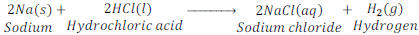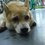# ACIDAcids ~ The word ‘Acid’ came from Latin word ‘Acidus or Acere’ which means sour.

~Sour taste is the most common characteristic of acid. ~ Acid turns blue litmus paper red. There are many substances which contain acid and hence taste sour, such as curd, tamarind, lemon, etc.

                                                Types of Acid


Natural Acid
~ Acids which are obtained from natural sources are called natural acid or organic acid.

Name of Acid Source Acetic acid Vinegar Ascorbic acid Amla Guava Citric acid Lemon Lactic acid Sour milk Methanoic acid Ant sting

Mineral Acids ~ Acids that prepare from mineral are known as mineral acids, inorganic acids, man-made acids or synthetic acid, such as hydrochloric acid, sulphuric acid, nitric acid, etc.

                                  Reaction of acids with metal:


Acids give hydrogen gas along with respective salt when they react with a metal.

METAL + ACID ------> SALT + HYDROGEN GAS

EXAMPLE:

~Hydrogen gas and zinc chloride are formed when hydrochloric acid reacts with zinc metal.

Zn + 2HCl -------> ZnCl2 + H2

                                                               QUIZ


Which of the following gives the correct increasing order of acidic strength?

(a) Water < Acetic acid < Hydrochloric acid

(b) Water < Hydrochloric acid < Acetic acid

(c) Acetic acid < Water < Hydrochloric acid

(d) Hydrochloric acid < Water < Acetic acidNote by Nicole Ling
7 years, 5 months ago

This discussion board is a place to discuss our Daily Challenges and the math and science related to those challenges. Explanations are more than just a solution — they should explain the steps and thinking strategies that you used to obtain the solution. Comments should further the discussion of math and science.

When posting on Brilliant:

• Use the emojis to react to an explanation, whether you're congratulating a job well done , or just really confused .
• Ask specific questions about the challenge or the steps in somebody's explanation. Well-posed questions can add a lot to the discussion, but posting "I don't understand!" doesn't help anyone.
• Try to contribute something new to the discussion, whether it is an extension, generalization or other idea related to the challenge.

MarkdownAppears as
*italics* or _italics_ italics
**bold** or __bold__ bold
- bulleted- list
• bulleted
• list
1. numbered2. list
1. numbered
2. list
Note: you must add a full line of space before and after lists for them to show up correctly
paragraph 1paragraph 2

paragraph 1

paragraph 2

[example link](https://brilliant.org)example link
> This is a quote
This is a quote
    # I indented these lines
# 4 spaces, and now they show
# up as a code block.

print "hello world"
# I indented these lines
# 4 spaces, and now they show
# up as a code block.

print "hello world"
MathAppears as
Remember to wrap math in $$ ... $$ or $ ... $ to ensure proper formatting.
2 \times 3 $2 \times 3$
2^{34} $2^{34}$
a_{i-1} $a_{i-1}$
\frac{2}{3} $\frac{2}{3}$
\sqrt{2} $\sqrt{2}$
\sum_{i=1}^3 $\sum_{i=1}^3$
\sin \theta $\sin \theta$
\boxed{123} $\boxed{123}$

Sort by:

Here's a bit of background context to acids and bases. Hope it won't be too long. ^^

So, let's start with water... If you leave water by itself, what might happen?

Maybe a water molecule comes and takes a hydrogen proton from another water molecule? Let me say that in another way...

2H$_2$O $\iff$ H$_3$O$^+$ + $^-$OH

There is some probability of this happening (i.e. H$_2$O disassociating itself into H$_3$O$^+$ and $^-$OH) and so scientists tend to take the [H$^+$] or [$^-$OH] concentrations of aqueous solutions and take the $\ -log_{10} [H^+] and [^-OH]$ and call them pH and pOH respectively.

Generally speaking, in chemistry, whenever you see a p in front of something, it means the $\ - log_{10}$ of that something. Also on a side note, the [H$^+$] concentration of water at 25$^ \circ$ celsius is 10$^{-7}$ and take the pH of that and you get 7.

And so, scientists care about what happens if something has a high H$^+$ concentration and less $^-$OH concentration and vice versa. Hence, we have a name for these and the name is... acids and bases!

Just to overcomplicate your life a little bit more (rofl), there are multiple definitions of acids and bases.

They are...

• Arrhenius $\Rightarrow$ Acids increase [H$^+$] in water and lower [$^-$OH] concentrations and bases vice versa.

For example, HCl + H$_2$O $\rightarrow$ H$_3$O$^+$ + Cl$^-$ and hydrochloric acid (or hydrogen chloride in aqueous solution) is a very strong acid (strong isn't just some nice word they use in chemistry, it literally means strong)... It completely disassociates itself, and notice the reaction was just one way. Not one of those wishy-washy equilibrium reactions, actually there is some small probability that it will go the other way but that's going into quantum mechanics.

Some examples of strong acids include HBr (Hydrogen bromide in aqueous solution or hydrobromic acid), HI (Hydriodic acid), HNO$_3$ (Nitric acid), H$_2$SO$_4$ (Sulfuric acid), HClO$_4$.

• Brønsted-Lowry $\Rightarrow$ Acid is a proton donor and a base is proton acceptor. I encourage you to check above, was HCl "donating" a H$^+$, and you will most likely say yes! This is just a broader definition. So all of Arrhenius acids and bases could also be Brønsted-Lowry acids and bases. Arrhenius acids deal with water and [H$^+$] concentration, but not all acids are like that.

For example, NH$_3$ + H$_2$O $\rightarrow$ NH$_4$OH, this donated a proton and it had nothing to do with water or [H$^+$] concentrations.

• Lewis $\Rightarrow$ The broadest definition, acid is an electron pair acceptor and a base is an electron pair donor. This definition is kinda squishy but, those examples above are considered a Lewis acid.

Example of a Lewis acid which is not a Brønsted-Lowry acid or an Arrhenius acid.

BF$_3$ + F$^-$ $\rightarrow$ BF$_4$, I know this doesn't make much sense but the boron trifluoride accepts an electron pair from the fluorine anion. Hence, BF$_3$ is a Lewis acid and F$^-$ is a Lewis base.

You'll come across mainly Arrhenius acids and bases in general chemistry, and that definition in my mind, is the easiest one to contemplate. Brønsted-Lowry acids/bases generally comes a lot in organic chemistry and Lewis acids/bases typically come in inorganic chemistry.

I was hoping it would be short but, oh well... I'm sorry, I got carried away.

Hope this is useful!~ ^^

- 7 years, 5 months ago

- 7 years, 5 months ago

My pleasure. ^^ Glad they're useful!

- 7 years, 5 months ago

I did this at 2:00-3:00 am, so I thought I might clarify some parts. Sorry in advance if you got confused. 0_o

Anyways, the [H$^+$] concentration in H$_2$O at 25$^\circ$ is 10$^{-7}\mathrm{M}$. That is $\mathrm{\frac {mol}{Litre}}$. Meaning, for every 1Litre of water, there is 10$^{-7}$ moles of [H$^+$] in the solution.

Also, HClO$_3$ is perchloric acid, which is an inorganic compound. Hope that clears some "future" doubts up. ^^

- 7 years, 5 months ago

Check out this acid called Carborane (HCB11Cl11) which has a ph level of -31. It has to be stored a special comtainers lined with Teflon to prevent it from melting the glass.

- 7 years, 5 months ago

Here is an interesting video on the chemistry of chili sauce!!! https://www.youtube.com/watch?v=U2DJN0gnuI8

- 7 years, 5 months ago

- 7 years, 5 months ago

yes. it's. :)

- 7 years, 5 months ago

A is correct

- 7 years, 5 months ago

yes. it's. :)

- 7 years, 5 months ago

A is right

- 7 years, 5 months ago

yes. it's. :)

- 7 years, 5 months ago

a is the ans

- 7 years, 5 months ago

yes. it's. :)

- 7 years, 5 months ago